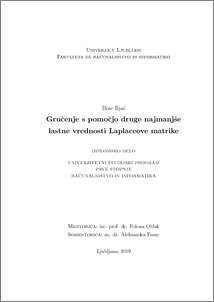# Clustering with the Second Smallest Eigenvalue of the Laplacian Matrix

Bine Iljaš (2019) Clustering with the Second Smallest Eigenvalue of the Laplacian Matrix. EngD thesis.Preview
PDF

## Abstract

In this work, the basic concepts of graphs and their corresponding matrices are discussed, as well as the Laplacian matrix and the properties of the eigenvector. The results demonstrate that the components of the Fiedler vector (vector corresponding to the second smallest eigenvalue of the Laplacian matrix), divide the graph into two groups. The following clustering methods are outlined: k-means, the spectral partitioning method and the modularity maximization method. An algorithm was implemented that uses k-means, or the recursive method, to divide the graph into subgroups. The experiment was carried out using artificial data as well as real data. The results are presented and compared with the method of maximizing the modularity. The Rand index is used to evaluate the success of the clustering. It is discovered that when using artificial data, all methods give comparable results, however using the method of maximizing the modularity gives slightly better results than the other two when using real data.

Item Type: Thesis (EngD thesis)
Keywords: Graphs, Laplacian matrix, Fiedler vector, clustering, k-means, modularity.
Number of Pages: 56
Language of Content: Slovenian
Mentor / Comentors:
Name and SurnameIDFunction
izr. prof. dr. Polona Oblak6760Mentor
as. dr. Aleksandra FrancComentor